# TCS Previous Year Question 2017

TECHNICAL

Certain questions are self-explanatory. Thus detailed solutions are provided only wherever required.

1. What the below statement will print if a=5? printf("%d %d",a, !a++);

a. 5 0 b. 6 0 c. 5 1 d. 6 1

Explanation

Values in the function get passed from right to left. First !a++ get processed which pass zero as argument and make a equal to 6

2. What is the output?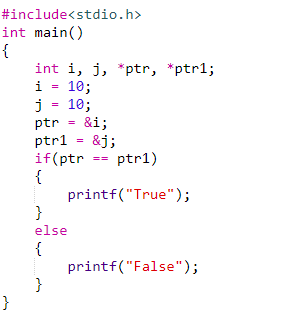a. 1 b. False c. Compiler Error d. No output

Explanation:

In this program, we are comparing the addresses contained by ptr & ptr1 not the value at those addresses and pointers ptr and ptr1 have the addresses of different variables so above condition is false

3. How many times main() will get called?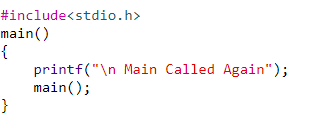a. Error b. Infinite times

c. No output d. None of these

Explanation:

There is no condition in the main() to stop the recursive calling of the  main()  hence it will be called infinite no of times.

4. Comment on the following?

const int *ptr;

a. You cannot change the value pointed by ptr

b. You can change the value of the pointer

c. None of these

d. You can change the pointer as well as the value pointed by it

Explanation:

Value of the pointer cannot be changed when const keyword is used

5. What is the output of the following program?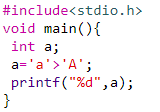a. NULL b. Error c. 0 d. 1

Explanation:

ASCII codes are compared. ’A’ is 65 while ’a’ is 97

6. What is the output of the following program?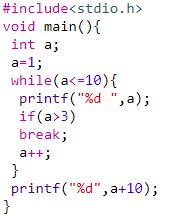a. 1 2 3 4 10 b. 1 2 3 4 14

c. 1 2 3 13 d. 1 2 3 14

Explanation:

1,2,3,4 are printed. When a value is greater than 4, if condition becomes true and break is executed. (4+10)14 is printed

7. Which of the following does not initialize ptr to null (assuming variable declaration of a as int a=0)?

a.int *ptr = &a; b. int *ptr = &a - &a;

c.int *ptr = a -a; d. None of these

Explanation:

In *ptr = &a, the address of a is stored in pointer. In other cases, it is 0

8. What is the output of the following program?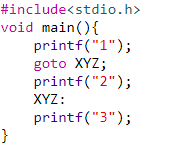a. 3 b.13 c. 123 d. 12

Explanation:

go to statement jumps program execution to the label XYZ and skips 2.

9. The statement that transfers control to the beginning of the loop is called _______.

a. Break statement b. Exit statement

c. Goto statement d. continue statement

Explanation:

Continue statement continues with the next iteration of the loop

10. What is the output of following program?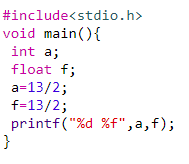a. 6 6.500000 b. 6 6.5

c. 6 6.000000 d. None of these

Explanation:

The solution of 13/2 is obtained as an integer and decimal values are dropped before assigning to f

# TCS Previous Year Question 2017

TECHNICAL

Certain questions are self-explanatory. Thus detailed solutions are provided only wherever required.

1. What is the purpose of ftell?

a. To get the current file name

b. To get the current file status

c. To get the current file attributes

d. To get the current file position

Explanation:

ftell() is used to find out the position of file pointer in the file with respect to starting of the file.

2. What will be the output of the below code?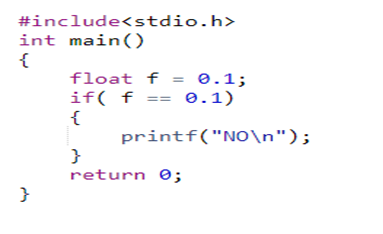a. NO output b. NO

c. Successfully complied but no output d. ERROR

Explanation:

No error and if condition fails so there is no output

3. What is the output of the below-mentioned programme?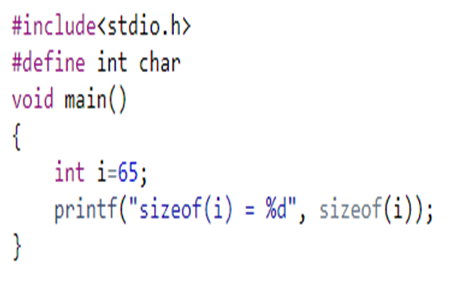a. sizeof(i) = 2 b. sizeof(i) = 1

c. compile Error d. None of these

Explanation:

int size is 2bytes or 4 bytes. Char size is 1 byte. Int is defined as Char. Sizeof(i) = 1

4. For passing command line argument the main function should be like

a. int main(int argc, char *argv[])

b. int main(int argc)

c. int main(char *argv[])

d. int main(char *argv[], int argc)

Explanation:

Command Line Arguments Syntax

5. Where the local variables are stored?

a. Disk b. Stack c. Heap d. OS

Explanation:

Local Variable is stored in stack

6. Which of the below functions is NOT declared in string.h?

a. strptr () b. strcpy ()

c. strlen() d. strupr()

Explanation:

strptr() is not declared

7. What is the output of the below-mentioned programme?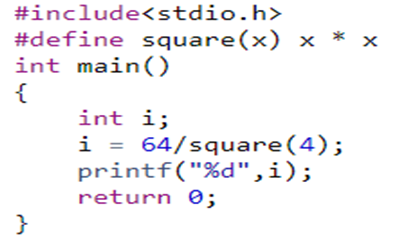a. 16 b. Compilation Error

c. 64 d. 4

Explanation:

It executes based on the operator precedence. 64 / 4 * 4.  16*4 = 64

8. What is the format of conditional operator?

a. Condition? true value: false value

b. Condition! true value: false value

c. Condition? false value: true value

d. Condition? true value: :false value

Explanation:

Syntax

9. What is recursion?

a. Looping

b. A function calls another function repeatedly

c. A function calls repeatedly

d. A function calls itself repeatedly

Explanation:

In recursion, the function calls itself repeatedly

10. A memory leak happens when?

a. A program allocates memory in heap but forget to delete it.

b. A program allocates memory in stack.

c. When an unsigned pointer is freed using free function.

d. When realloc() is called on a pointer that is not allocated

Explanation:

A memory leak occurs when programmers create a memory in heap and forget to delete it.